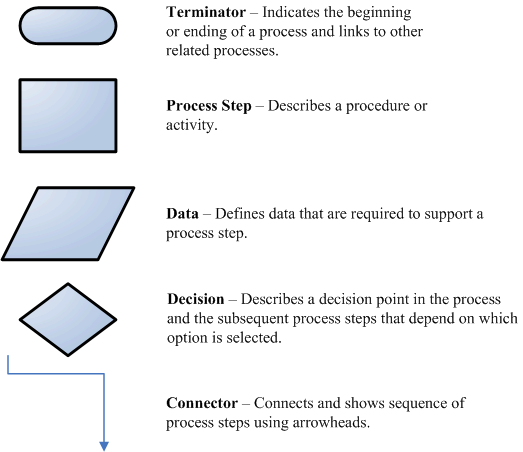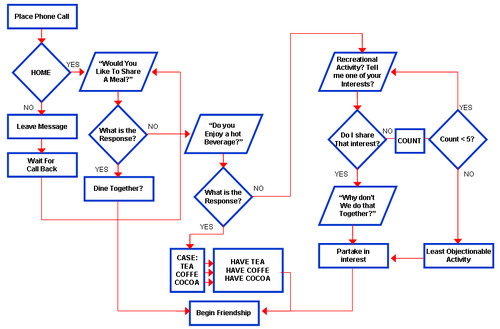# Class 4 Notes for Webscripting

## Learning Objectives:

You will be able to create an array

You will be able to create a switch

You will be able to identify the use of conditional statements.

## Homework:

Create: Flow Chart for buying a comptuer or a car and Link to Homework page!

Watch: How to do a Flow Chart

Watch: Big Bang Theory Friendship Algorith shows why you do a flow chart.

##Topics Covered:

Watch a flow chart in action

Array is a variable on steroids. (Array's have several values under the same variable name.) You have to declare an array!

var mynewarray= new Array()

mynewarray, mynewarray,mynewarray Notice the array number starts with 0.

Conditional Statements (Java script's method of testing the environment)

if statement - use this statement if you want to execute some code only if a specified condition is true

if (condition)
{ code to be executed if condition is true }

if...else statement - use this statement if you want to execute some code if the condition is true and another code if the condition is falseif (condition)
{ code to be executed if condition is true }

else
{ code to be executed if first condition is not true }

if...else if....else statement - use this statement if you want to select one of many blocks of code to be executed - >Note: You may want to use Switch if you have more than 3 conditions
Syntax

if (first_condition)
{ code to be executed if condition is true }

else if (second_condition)
{ code to be executed if first condition is not true and this condition is true}

else
{ code to be executed if 1st & 2nd condition is not true }

switch statement - use this statement if you want to select one of many blocks of code to be executed

switch(n) {

case 1:

execute code block 1

break

case 2:

execute code block 2

break

default:

code to be executed if n is different from case 1 and 2

}

Two different kind of loops In JavaScript, there are:

1. for - loops through a block of code a specified number of times

for (variable=startvalue;variable<endvalue;variable=variable+increment)
{
code block
}

2. while - loops through a block of code while a specified condition is true

while (variable<endvalue)
{
code block
}

:

## Logical Operators

Operator Description Example
&& and x=9
y=4

(x < 10 && y > 1) returns true

|| or x=6
y=3

(x==5 || y==5) returns false

! not x=10
y=5

!(x==y) returns true

## Terms:

Flow Charts, Conditional Statements, if else, switch, Function, Operators and Argument